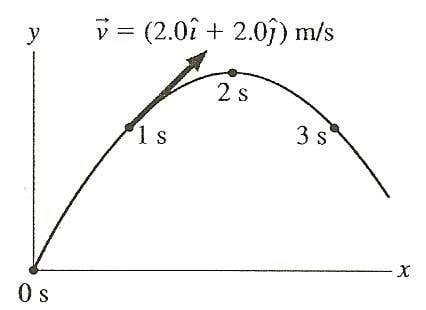# Basic Projectile Motion Problem

## Homework Statement

A physics student on PLanet Exidor throws a ball, and it follows hte parabolic trajectgory shown in the figure. The ball's position is shown at 1.00 intervals until t=3.00. At t=1.00, the ball's velocity is v=(2.00i + 2.00j)[m/s].a. Determine the ball's velocity at t=0, 2.00, and 3.00.
b. What is the value of g on the Planet Exidor?
c. What was the ball's launch angle?

$$v = v_0 + a t [tex] [tex] x = x_0 + v_0 t + (1/2) a t^2 [tex] [tex] v^2 = v_0^2 + 2 a \Delta x [tex] ## The Attempt at a Solution I was able to find at 1.00 that the ball's acceleration in the x direction = 2.00[m/s^2]. I'm not really sure how to find the velocity at t=0 since I think it can't be zero. I'm pretty if I can figure out the max height I can figure out what g is. But I can't find max height. Thanks. ## Homework Statement ## Homework Equations ## The Attempt at a Solution ## Answers and Replies Kurdt Staff Emeritus Science Advisor Gold Member With all projectile motion problems you need to consider both components of motion. There should be no acceleration in the x direction. At the top of the parabola what do you expect the y component of velocity to be? This will help you with what you know about t = 1 to set up some simultaneous equations and solve the problem. There should be no acceleration in the x direction. It will really help to rewrite [tex] v = v_0 + at = v_0 - gt$$

where i've defined g downwards as positive as two equations then...

$$v_x = ... \quad \& \quad v_y = v_y(t)=...$$

Thanks for the help. It was stupid of me to think of ax instead of just thinking g the whole time. This clarified things a bunch, thanks!